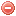# Description

The Munger index was developed in Oregon (USA) at the beginning of the 20th century. It is a very simple fire only requiring precipitation as input data. As the relationships between most meteorological factors influencing fire hazard (wind, precipitation, air humidity, etc.) were considered too complex, and as the most relevant factor in the Pacific Northwest was considered to be the intensity of drought periods, Munger (1916) proposed a simple drought index based on the length of periods without rainfall, assuming that the relationship between drought length and drought intensity was not linear.

The purpose of this index was to easily identify and graphically represent drought intensity, and to allow the comparison of fire risk during different years or in different regions.

The Munger index resets to zero when daily precipitation exceeds 0.05 inch (about 1.27 mm) and particularly appropriate for measuring short-term drought (Heim 2002). Similarly to the Nesterov index, it might therefore be suitable for capturing fine fuel moisture and thus predict ignition, but less suitable for predicting fire behavior or spread.

While this index has not been much used for forest fire research or management, it has sometimes been used for assessing drought severity in agriculture or hydrology (cf. Heim 2002)

# Formula

The Munger index is calculated as follows:

$\mathit{Munger}=\dfrac{1}{2}\cdot{w^2}$

where $$w$$ is the number of days since the last daily rainfall greater than 0.05 inch

The Munger index is supposed to be calculated on a daily basis.

Wet conditions are required for starting the index calculation (daily rainfall > 0.05 [in] on the day preceeding the start of the calculation). The starting value has to be set to 0.

Original publication:
Munger (1916)

### Bibliography SearchNo results!

No results were found. Are you sure you searched for a tag?

### Symbols

 Variable Description Unit $$T$$ air temperature °C $$T_{dew}$$ dew point temperature °C $$H$$ air humidity % $$P$$ rainfall mm $$U$$ windspeed m/s $$w$$ days since last rain (or rain above threshold) d $$rr$$ days with consecutive rain d $$\Delta t$$ time increment d $$\Delta{e}$$ vapor pressure deficit kPa $$e_s$$ saturation vapor pressure kPa $$e_a$$ actual vapor pressure kPa $$p_{atm}$$ atmospheric pressure kPa $$PET$$ potential evapotranspiration mm/d $$r$$ soil water reserve mm $$r_s$$ surface water reserve mm $$EMC$$ equilibrium moisture content % $$DF$$ drought factor - $$N$$ daylight hours hr $$D$$ weighted 24-hr average moisture condition hr $$\omega$$ sunset hour angle rad $$\delta$$ solar declination rad $$\varphi$$ latitude rad $$Cc$$ cloud cover Okta $$J$$ day of the year (1..365/366) - $$I$$ heat index - $$R_n$$ net radiation MJ⋅m-2⋅d-1 $$R_a$$ daily extraterrestrial radiation MJ⋅m-2⋅d-1 $$R_s$$ solar radiation MJ⋅m-2⋅d-1 $$R_{so}$$ clear-sky solar radiation MJ⋅m-2⋅d-1 $$R_{ns}$$ net shortwave radiation MJ⋅m-2⋅d-1 $$R_{nl}$$ net longwave radiation MJ⋅m-2⋅d-1 $$\lambda$$ latent heat of vaporization MJ/kg $$z$$ elevation m a.s.l. $$d_r$$ inverse relative distance Earth-Sun - $$\alpha$$ albedo or canopy reflection coefficient - $$\Delta$$ slope of the saturation vapor pressure curve kPa/°C $$Cc$$ cloud cover eights $$ROS$$ rate of spread m/h $$RSF$$ rate of spread factor - $$WF$$ wind factor - $$WRF$$ water reserve factor - $$FH$$ false relative humidity - $$FAF$$ fuel availability factor - $$PC$$ phenological coefficient -

 Suffix Description $$-$$ mean / daily value $$_{max}$$ maximum value $$_{min}$$ minimum value $$_{12}$$ value at 12:00 $$_{13}$$ value at 13:00 $$_{15}$$ value at 15:00 $$_{m}$$ montly value $$_{y}$$ yearly value $$_{f/a}$$ value at fuel-atmosphere interface $$_{dur}$$ duration $$_{soil}$$ value at soil level

 Constant Description $$e$$ Euler's number $$\gamma$$ psychrometric constant $$G_{SC}$$ solar constant $$\sigma$$ Stefan-Bolzmann constant